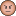# New to Qlik Sense

If you’re new to Qlik Sense, start with this Discussion Board and get up-to-speed quickly.

Announcements
Qlik Insider - Maximize your investment, Join this webinar, May 26th. Register Today
cancel
Showing results for
Did you mean:Contributor II

## Set Analysis based on Rank

Hi,

I'm struggling with this issue for several days.

I want to calculate the Total value of the N customers with the same conditions.

Meaning, first calculate the total sale per Customer with some conditions (set analysis), then rank them, and finally sum the total sales for the first N top customer. the N is a variable the can be changed by the user.

somthing like this

sum(<{Field1={''},field2={''}, Customer = { Rank( sum(< {Field1={''},field2={''} > Sales } <= \$(NTopCustomer))} Sales)

thanks1 Solution

Accepted SolutionsMVP

Try this

Sum({<Field1 = {''}, field2 = {''}, Customer = {"=Rank(Sum({<Field1 = {''}, field2 = {''}>} Sales)) <= \$(NTopCustomer)"}>} Sales)

15 RepliesMVP

Try this

Sum({<Field1 = {''}, field2 = {''}, Customer = {"=Rank(Sum({<Field1 = {''}, field2 = {''}>} Sales)) <= \$(NTopCustomer)"}>} Sales)Contributor II
Author

at first, it didn't work, but I found out what was the problem

one of my conditions is based on a variable

[DATE.autoCalendar.QuartersAgo]={"<=\$(DateRangeSelection)"}MVP

What are Field1 and field2? Are these placeholders for your actual fields or that is what they are called? If they are the real field names, make sure you have the correct casing for them... meaning is field2 called Field2? or may be Field1 is called field1...

There could be many things which could be not right... difficult to say what might be issue more details. Are you able to share a sample if what I mentioned above is not an issueContributor II
Author

my conditions are

[DATE.autoCalendar.QuartersAgo]={"<=\$(DateRangeSelection)"} , [CHANNEL]={'DIRECT'}MVP

May be try one of these

[DATE.autoCalendar.QuartersAgo]={"<=\$(=DateRangeSelection)"}

or

[DATE.autoCalendar.QuartersAgo]={"<=\$(=\$(DateRangeSelection))"}Contributor II
Author

nope still -MVP

How do you define DateRangeSelection?Contributor II
Author

it's working in the set analysis outside the Rank

it's a variable that gets the value from the extension "Variable Toggle" and the options are:   0 , 3 , 7MVP

Have you checked that the variable actually takes a value once you set it using your extension? Check the variable overview and see what value do you see for DateRangeSelection variable